Next: Stability Equations Up: Coupled Oscillations Previous: Introduction

Equilibrium State

Consider an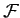degree of freedom dynamical system described by the generalized coordinates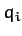, for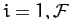. Suppose that the kinetic energyand the potential energy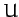are not explicit functions of time. This implies that the system in question is isolated: i.e., it is not subject to any external forces or time-varying constraints. In virtually all dynamical systems of interest, the kinetic energy can be expressed as a quadratic form: i.e.,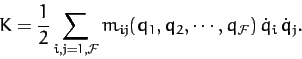(770)

Without loss of generality, we can specify that the weights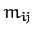in the above form are invariant under interchange of the indicesand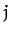: i.e.,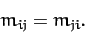(771)

Finally, the potential energy is written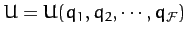.

Suppose that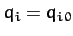, for, corresponds to an equilibrium state of the system. It follows thatand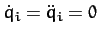, for, should be a possible solution of the equations of motion. Now, Lagrange's equations of motion for the system take the form [see Equation (613)]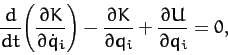(772)

for. Here, we have made use of the definition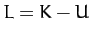, and the fact thatis independent of the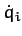. Now, it is clear, from an examination of Equation (770), that every component making up the first two terms in the above equation contains either a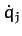or a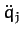, for some. But, we can set all of the generalized velocities and accelerations to zero in an equilibrium state of the system. Hence, the first two terms in the above equation are zero, and the condition for equilibrium reduces to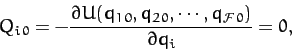(773)

for. In other words,, for, is an equilibrium state provided that all of the generalized forces,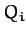[see Equation (599)], evaluated at, are zero. Let us suppose that this is the case.Next: Stability Equations Up: Coupled Oscillations Previous: Introduction
Richard Fitzpatrick 2011-03-31